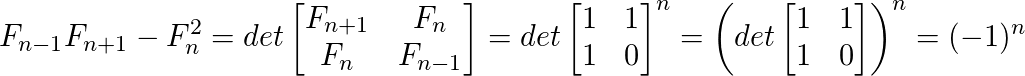GeeksforGeeks App
Open AppBrowser
Continue

## Related Articles

• Write an Interview Experience
• Mathematical Algorithms

# Cassini’s Identity

Given a number N, the task is to evaluate below expression. Expected time complexity is O(1).

` f(n-1)*f(n+1) - f(n)*f(n)`

Where f(n) is the n-th Fibonacci number with n >= 1. First few Fibonacci numbers are 0, 1, 1, 2, 3, 5, 8, 13, ………..i.e. (considering 0 as 0th Fibonacci number) Examples :

```Input : n = 5
Output : -1
f(5-1=4) = 3
f(5+1=6) = 8
f(5)*f(5)= 5*5 = 25
f(4)*f(6)- f(5)*f(5)= 24-25= -1```

Although the task is simple i.e. find n-1th, nth and (n+1)-th Fibonacci numbers. Evaluate the expression and display the result. But this can be done in O(1) time using Cassini’s Identity which states that:

`           f(n-1)*f(n+1) - f(n*n) = (-1)^n `

So, we don’t need to calculate any Fibonacci term,the only thing is to check whether n is even or odd. How does above formula work? The formula is based on matrix representation of Fibonacci numbers.C/C++

` `

## C++

 `// C++ implementation to demonstrate working``// of Cassini’s Identity``#include ``using` `namespace` `std;` `// Returns (-1)^n``int` `cassini(``int` `n) { ``return` `(n & 1) != 0 ? -1 : 1; }` `// Driver Method``int` `main()``{``    ``int` `n = 5;``    ``cout << (cassini(n));` `    ``return` `0;``}` `// This code is contributed by phasing17`

## Java

 `// Java implementation to demonstrate working``// of Cassini’s Identity` `class` `Gfg``{``    ``// Returns (-1)^n``    ``static` `int` `cassini(``int` `n)``    ``{``       ``return` `(n & ``1``) != ``0` `? -``1` `: ``1``;``    ``}` `    ``// Driver method``    ``public` `static` `void` `main(String args[])``    ``{``         ``int` `n = ``5``;``         ``System.out.println(cassini(n));``    ``}``}`

## Python3

 `# Python implementation``# to demonstrate working``# of Cassini’s Identity` `# Returns (-1)^n``def` `cassini(n):` `   ``return` `-``1` `if` `(n & ``1``) ``else` `1`` ` `# Driver program`` ` `n ``=` `5``print``(cassini(n))``   ` `# This code is contributed``# by Anant Agarwal.`

## C#

 `// C# implementation to demonstrate``// working of Cassini’s Identity``using` `System;` `class` `GFG {` `    ``// Returns (-1) ^ n``    ``static` `int` `cassini(``int` `n)``    ``{``       ``return` `(n & 1) != 0 ? -1 : 1;``    ``}`` ` `    ``// Driver Code``    ``public` `static` `void` `Main()``    ``{``         ``int` `n = 5;``         ``Console.Write(cassini(n));``    ``}``}` `// This code is contributed by Nitin Mittal.`

## PHP

 ``

## JavaScript

 ``

Output :

`-1`

Time complexity: O(1) since only constant operations are performed

Auxiliary Space: O(1)